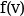Scilab Home page | Wiki | Bug tracker | Forge | Mailing list archives | ATOMS | File exchange
Change language to: Français - Português - 日本語 - Русский
Scilab Help >> Differential calculus, Integration > integrate

integrate

integration of an expression by quadrature

Syntax

x=integrate(expr,v,x0,x1 [,atol [,rtol]])

Arguments

expr

a character string defining a Scilab expression.

v

a character string, the integration variable name.

x0

a real number, the lower bound of integration.

x1

a vector of real numbers, upper bounds of integration.

atol

a real number (absolute error bound). Default value: 1e-8.

rtol

a real number, (relative error bound). Default value: 1e-14.

x

a vector of real numbers, the integral value for each x1(i).

Description

x=integrate(expr,v,x0,x1 [,atol [,rtol]]) computes :for i=1:size(x1,'*') whereis given by the expression expr.

The evaluation hopefully satisfies following claim for accuracy: abs(I-x)<= max(atol,rtol*abs(I)) where I stands for the exact value of the integral.

Restriction

The given expression should not use variable names with a leading %.

Examples

x0=0;x1=0:0.1:2*%pi;
X=integrate('sin(x)','x',x0,x1);
norm(cos(x1)-(1-X))

x1=-10:0.1:10;
X=integrate(['if x==0 then 1,';
'else sin(x)/x,end'],'x',0,x1)

• intg — definite integral
• inttrap — integration of experimental data by trapezoidal interpolation
• intsplin — integration of experimental data by spline interpolation
• ode — ordinary differential equation solver